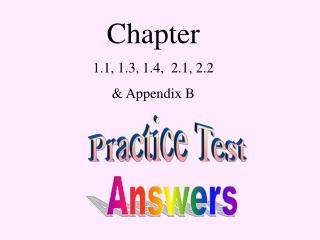DownloadDownload PresentationPractice Test

# Practice Test

Télécharger la présentation## Practice Test

- - - - - - - - - - - - - - - - - - - - - - - - - - - E N D - - - - - - - - - - - - - - - - - - - - - - - - - - -
##### Presentation Transcript

1. Chapter 1.1, 1.3, 1.4, 2.1, 2.2 & Appendix B Practice Test Answers

2. #1

3. Simplify Multiply top and bottom by -1

4. Simplify Multiply top and bottom by -1 1

5. Original Function

6. COMPARE ? YES EVEN

7. #2

8. To prepare the right for completing the square Result Factor out –1/3

9. To prepare the right for completing the square Result Factor out –1/3 ?

10. Continued Add to complete the square What did we actually add to the right side ?

11. Continued Add to complete the square

12. Continued Add to complete the square Need to add it to the left side also.

13. Continued Add to complete the square Result

14. Continued Represent the perfect square as a binomial squared. Isolate y

15. Vertex

16. #3

17. Vertex

18. Vertex

19. Vertex Axis of Symmetry

20. Vertex Axis of Symmetry

21. Vertex y-intercept Axis of Symmetry

22. X-intercept(s) Vertex Axis of Symmetry y-intercept

23. X-intercept(s) Vertex Axis of Symmetry Factor y-intercept

24. X-intercept(s) Vertex Axis of Symmetry y-intercept Solve

25. X-intercept(s) Vertex Axis of Symmetry y-intercept

26. X-intercept(s) Vertex Axis of Symmetry y-intercept

27. #4

28. Determine whether the graph of the equation below is symmetric with respect to the y-axis, x-axis, origin, the line y = x or none of these. y-axis –x for x Equivalent? YES

29. y-axis –x for x Equivalent? YES Determine whether the graph of the equation below is symmetric with respect to the y-axis, x-axis, origin, the line y = x or none of these. x-axis –y for y Equivalent? YES

30. y-axis x-axis –x for x –y for y Equivalent? Equivalent? YES YES Determine whether the graph of the equation below is symmetric with respect to the y-axis, x-axis, origin, the line y = x or none of these. origin –x for x –y for y Equivalent? YES

31. Determine whether the graph of the equation below is symmetric with respect to the y-axis, x-axis, origin, the line y = x or none of these. y-axis The line y = x YES Trade x for y x-axis YES origin Equivalent? NO YES

32. Determine whether the graph of the equation below is symmetric with respect to the y-axis, x-axis, origin, the line y = x or none of these. y-axis The line y = x YES NO x-axis YES origin YES

33. #5